Roger's Equations Blog### Roger's Equations

This blog is all about science and technology (with occasional math thrown in for fun). The goal of this blog is to try and pass on the sense of excitement and wonder I feel when I read about these topics. I hope you enjoy the posts.

 Previous in Blog: Global Warming (Part 3 of 3) Next in Blog: Quadratic Formula and Cubic Formula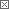# Deriving the Speed of Light

Posted September 14, 2006 4:26 PM by Bayes

The fact that the speed of light is constant regardless of your reference frame is a direct consequence of Maxwell's Equations. Einstein used this peculiar feature of light as the basis for special relativity. So how does the speed of light appear from Maxwell's Equations?

Starting with Maxwell's equation for the curl of an electric field:Take the curl of both sides,using the vector identity:and Maxwell's equation for an Electric Field in a Vacuum (no charge):We see that the Electric Field Wave equation is:where,This differential equation is in the form of the wave equation: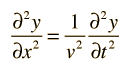This differential equation describes a sinusoidal wave propagating in the x-direction with velocity V.

With solutions: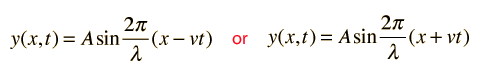So the solution to the Electric Field Wave Equation is:

## E=E0Sin(kx-±ωt)

Where ω (the angular frequency) is V/K and K (the wavenumber) is 2π/λ, here V is the velocity of the wave and λ is it's wavelength.

So taking another look at the Electric Field wave equation:We see that the Electric Field is a sinusodial wave propagating at speed c. With a similiar method we can derive a Magnetic Field wave equation which describes the magnetic field as a sinusoidal wave propagating with speed c. So light, which is an Electromagnetic Wave, propagates with speed c.

Special Thanks to Wikipedia for the Equations.

Interested in this topic? By joining CR4 you can "subscribe" to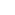#1

###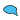Re: Deriving the Speed of Light

09/15/2006 2:17 AM

Hi Roger,

It's a very nice summary of the origins of c - thanks!

It did however take Einstein and his insights on simultaneity and synchronization of clocks to make the independence of c from the frame of reference understandable.

__________________
"Perplexity is the beginning of knowledge." -- Kahlil Gibran#2

###Re: Deriving the Speed of Light

09/19/2006 11:29 AM

I agree with you. All the derivation above showed was where the speed limit for light comes from. In the end,

c=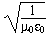.

Whats interesting is what this tells us. ε0 is the Electric Permitivity and μ0 is the Magnetic Permeability of free space. ε0 is constant, but if it was larger, then the speed of light would be slower. Also the case with μ0. So what does a larger ε0 mean? Well, The Coulomb force is F= 1/4πε0 (Qq/r2). This means as ε0 increases, the Force decreases. So essentially, the stronger the electromagnetic force is, the smaller ε0 and μ0 are, the faster the speed of light. Of course, the electromagnetic force doesn't change (as far as we know) and ε0 and μ0 are constant.

HOWEVER, once in a while some particle physicists will mention that the fine constant may have changed over time. This implies that the Electromagnetic Force has been stronger in the past and light was faster. I'm not sure I'm ready to believe anything like that, but it is mentioned.

Active Contributor

Join Date: Aug 2006
Posts: 14#3

###Re: Deriving the Speed of Light

09/19/2006 8:09 PM

I appreciate your taking the time to educate us in the origins of 'standard' equations and rules. One thing I have always wanted to see is the proof for 1ˆ0=1 (or any real number raised to the power of zero equaling one).

If you ever run out of ideas or get the time, I think it would make a good post.

__________________
No electrons were harmed in the making of this message,(although a few hundred billion were temporally inconvenienced)
Associate

Join Date: Aug 2005
Posts: 54#4

###Re: Deriving the Speed of Light

09/20/2006 6:50 AM

I think the logarithmic rule should able to explain this.

Logarithm of a Number A is the number of times the base is raised (the power)to give the number.

Illustration: Log 2 4 = 2 i.e 2 2 = 4

Let log 2 1 = X …… (1)

Log 3 1 = Y ……(2)

Log 4 1 = Z ……..(3)

Therefore; 2X = 1 ……(4)

3Y =1 …….(5)

4Z = 1 …….(6)

Equating eqns (4)(5)(6); 2X = 3Y = 4Z = 1

i.e x = y = z = 0.

Any number raised to power of zero should be equal 1 (one)#5

###Re: Deriving the Speed of Light

09/20/2006 7:31 AM

Another very simple way of looking at it is this: plot the equation y = ax with a being any constant larger than 1 and vary x linearly from -1 to 1. The curve will vary exponentially from y=1/a to y=a and always cut the y-axis at y=1; therefore a0=1.

__________________
"Perplexity is the beginning of knowledge." -- Kahlil Gibran#6

###Re: Deriving the Speed of Light

09/20/2006 10:57 AM

KasterKid,

Thanks for the suggestion! I'll put it on the list for a future post (It may be a few weeks from now). I'm not sure I'll be able to offer a proof, but I'll try to make a convincing case by approaching the problem from different directions (series expansions, graphing, algebra, etc.)

Power-User

Join Date: Mar 2006
Location: UK
Posts: 129#9

###Re: Deriving the Speed of Light

09/25/2006 3:56 PM

For any number N, Nx1=N, so N/N =1

N = N^1

NxN = N^2

NxNxN =N^3

N/N = N^0

It is just a question of defining N to the power of zero
All jargon is invented to simplify communication for its users but almost all of it tends to baffle those without a translator#10

###Re: Deriving the Speed of Light

09/26/2006 3:10 PM

You wrote"It is just a question of defining N to the power of zero. All jargon is invented to simplify communication for its users"

I don't agree. Math is very special because as a way of describing things, it has to be self consistent. The beauty of math is, you need only show something doesn't work in one way to prove it wrong. Take your example above, you wrote:

N/N = N^0 where N can be any number other than zero

This isn't true only because you defined it to be true, it's true because its consistent within the greater framework of math as a whole.

Lets see what happens when we test your "definition"; Since N represents any number, it's perfectly ok to replace N with X (which I'm doing only for aesthetic purposes).

x/x = x0= 1

and furthermore, there should be no problem with me taking the derivative with respect to x on both sides;

d/dx(x/x)=d/dx(x0)=d/dx(1)

which gives the equation

1/x - 1/x = 0 = 0, or

0 = 0 = 0.

Also we can just multiply the exponents;

1=x/x=x0 is the same as

1=(x+1)(x-1)=x0

noting

(xn)(xm)=x(n+m)

gives us

1=x0=x0

Not that I think for a second that I'm telling you anything you don't know here, I'm just trying to illustrate that it is not enough to simply define something in order for it to be true in math, it also has to fit into the existing framework completely, otherwise it cannot be used.

Power-User

Join Date: Mar 2006
Location: UK
Posts: 129#11

###Re: Deriving the Speed of Light

09/27/2006 9:16 AM

Those two sentences were in different paragraphs. I shouldn't dream of saying that maths is jargon although many mathematicians do use jargon occasionally (including myself, even though I decry it).

Of course you are right to use x - I chose to illustrate with N because a lot of people find it very easy to divide by an integer but more difficult to divide by a non-integer and hard to divide by an irrational number; it doesn't make any difference when you're dividing it into itself but it still feels easier.

Incidentally, while maths has to be self-consistent, I shouldn't claim that it is self-consistency that makes it special: I regard its self-consistency as an automatic side-effect of being RIGHT, but it is also possible to be self-consistent and wrong. Inconsistency is just one way to recognise and filter out the more obvious errors.
Mathematics provides a means of describing everything in the physical universe and in so doing helps us to understand things a bit better.#12

###Re: Deriving the Speed of Light

09/27/2006 9:29 AM

I agree with everything you said above. To clarify, when I say math is special because its self consistent, I meant it is a self consistent philosophy. There have been many different approaches to trying to describe the world we live in, but math and science have been the most successful because they demand self consistency.

There is a misconception that math is how the universe works. Actually, math is how we describe how the universe works. Just as writing is how we express what we are saying. Math is a tool, not an underlying truth, which can be seen in the way we are constantly refining it to handle new problems. Adding zero, infinity, i, etc. have given us the opportunity to describe things in greater detail, and there is no doubt that future additions will provide us with more ways to describe things.

Anonymous Poster#13

###Re: Deriving the Speed of Light

07/28/2008 6:45 PM

The simplest way is for x a real number,

1=(x^1)/(x^1) =x^(1-1) =x^0

Mathman633

Anonymous Poster#15

###Re: Deriving the Speed of Light

03/18/2010 4:05 PM

0=x-x for any nonzero number x

now put it at the place of and do maths for the powers as normal ie 1^x/1^x which comes out to be 1 even doesn't matter whether 1 or 2 but not zero.

that's why 0^0 isn't defined......

i think you got the point.

this is index method

Active Contributor

Join Date: Jun 2006
Location: Richmond, Virginia
Posts: 13#7

###Re: Deriving the Speed of Light

09/22/2006 1:21 AM

I do not think the speed of light is constant. This is why. Nothing escapes a black hole. Correct? The only reason we can "see" a black hole is because of the absence on light. Now with gravity it gets stronger the closer you get to the object (ie. stars, planets, black holes). now say we have a light particle passing by a black hole, at such a distance that, lets say 20% of it's gravity is present (or felt) at most. this I do believe causing a bending of light. So our little particle turns. Isn't turning, the slowing down of one side (and the subsequnit speeding up of the other side). Now, granted, this is on the scale of a particle. But dosen't a particle have dementions?

Now to change gears some. Same senario, but our light particle has intercepts a black hole at a angle. and our particle gets trapped by the black hole's gravity. Threoreticly, a light particle could orbit a black hole. Now just like when they send a space probe/craft out. They "sling shot" it using a planets gravity to increase the velocity of the probe/craft. Wouldn't our light particle experience the same effect?

Now I do not have a degree in any of this, so I can not back this up with formulas or anything else. But I do pose the question for disscussion.

__________________
There are 10 types of people in the world. Those who understand Binary and those who do not.#8

###Re: Deriving the Speed of Light

09/22/2006 2:16 AM

JustSimplyBob, you are partially right and partially wrong. According to Einstein, light's speed is constant for every inertial observer, when measured at that observer's location, even if it is very close to a black hole.

If a 'distant' observer could measure that same set of photons, he/she would observe a bending and a 'slowing down' of the photon stream near the black hole - scientists hate this 'slowing down' statement, but for us engineers it's (maybe) OK! The 'slowing down' of light manifests itself as the Shapiro time delay of light. The closer to the black hole the beam passes, the more the bending and the time delay. You can read more about this is a download from this page: Tests of Relativity.

__________________
"Perplexity is the beginning of knowledge." -- Kahlil Gibran
Participant

Join Date: Jun 2009
Posts: 1#14

###Re: Deriving the Speed of Light

06/21/2009 1:21 AM

Hi

I noticed in your blog entry that you state, since light satisifes the differential equation, thus it must be a sinusoidal wave. However, a sinusoid is one of many possible solutions to the wave equation. In fact in one dimension, any function of the form F(x-ct) will solve the wave equation. In general, light waves are not sinusoidal.